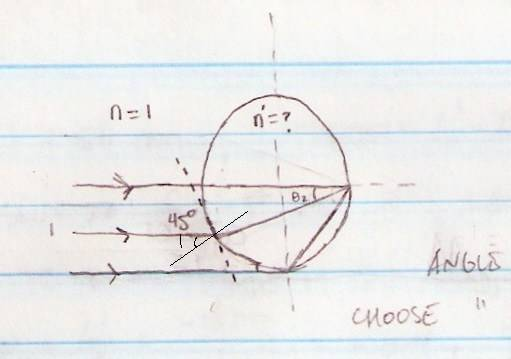# Finding index of refraction

Aelo

## Homework Statement

Consider a special crystal sphere, where light from a distant object is focused by the front surface of the sphere exactly onto the opposite side of the sphere. What is the index of refraction of the sphere? Assume the sphere is in air.

## Homework Equations

(n'/l') - (n/l) = (1/R)*(n' - n)
f' = n'/K = n'F

## The Attempt at a Solution

I know that n is taken to be 1 since the sphere is in air. Since the object is described to be distant, I'm guessing that perhaps l could be infinity. I've thought about trying to relate different angles to each other or looking at the sag, but it looks like the question is asking for a numerical value, and I don't know how to get there with what was given. Because it's focusing at the side of the sphere, we may be able to relate the radius to the focal point?

Last edited:

## Answers and Replies

Gold Member
Since the light is focused on the opposite face you know that the focal length is 2R; you also know that the radius of curvature is R. There is a great deal of symmetry.

You should be able to trace a single ray and then solve for n.

Aelo
Since f = -nF, I know that F = -2R. Similarly, f' = n'F. How do I find f'? Or am I over-simplifying it?

Gold Member
You don't need any of the lens equations; you already know where all of the rays terminate ... because your light is from a "distant source" the incoming rays are parallel, so assume that they illuminate one full hemisphere.

You can imagine the sphere as a globe, with the light illuminating the southern hemisphere - then the longest ray (and the only straight ray) travels from the south pole to the north pole, a distance of 2R, and the angle of incidence with the normal is 0. The rays hitting at the equator (just barely!) are at 90 degrees ... but these may not make the transit ... so pick a latitude close to the south pole (latitude theta-1), determine the angle of incidence all around this latitude circle, and the internal angle required to send each of these rays to the north pole (angle theta-2).

Now apply Snell's law to these two angles ... the geometric conditions will provide enough constraints to determine the index of refraction - and also the greatest circle of latitude which contributes to the light at the north pole!

Aelo
Why wouldn't the ray hitting at 90 degrees be usable? If I'm understanding you correctly, what I need to do is consider just the ray hitting at (or around) 90 degrees, find the angle of refraction once that ray has entered into the sphere, and say that n*sin(90 or almost 90) = n'*sin(angle of refraction).

Gold Member
If the equatorial ray makes it to the north pole then what is the angle of refraction?

Plug these angles into Snell's law and you will have a value for n' of the glass. But sin (90)=1, and n for air is 1, so n' would have to be 1/sin (45)=sqrt (2). So unless n'=1.414 the equatorial ray will not reach the pole. This does seem to match the problem statement.

Aelo
I thought this sphere was special in that every ray hitting it was focused to the sphere's opposite side. Wouldn't that mean that the equatorial ray would have to make it?

Gold Member
Just test another latitude, 45 degrees would work and see if n' is the same.

Aelo
What trig relationship should I use to find the value of the refracted ray?

Gold Member
The incident ray comes in parallel to the polar axis; the angle of incidence is thus the angle between the polar axis and the normal to the surface where the ray strikes ... if it strikes at latitude 45 the angle is 45. If it strikes at latitude 30 it is 60 ... draw a diagram of a circle to test this.

Then connect the ray from the point of incidence to the north pole ... the interior triangle made from the point of incidence and the two poles (draw it!) obeys a simple theorem from plane geometry. This will give the angle of refraction.

Aelo
I've had a drawing since the start, I've just been too lazy to post it. :uhh:

Here it is:Knowing only one angle of the three sides, I don't know how to find theta 2. Geometry was never a strength, unfortunately. It's not a right triangle, so I can't say that the sum of the 3 angles is 180.

Last edited:
Aelo
Looking at that and this: http://www.regentsprep.org/Regents/math/geometry/GP15/CircleAngles.htm

Wouldn't that indicate the upper left corner angle is 90 degrees, so that theta 2 = 180 - 90 - 45?